Скачать презентацию Problem 12 10 Determine the current in the

Problem-Solved-Three-Phase+Circuits.ppt

• Количество слайдов: 8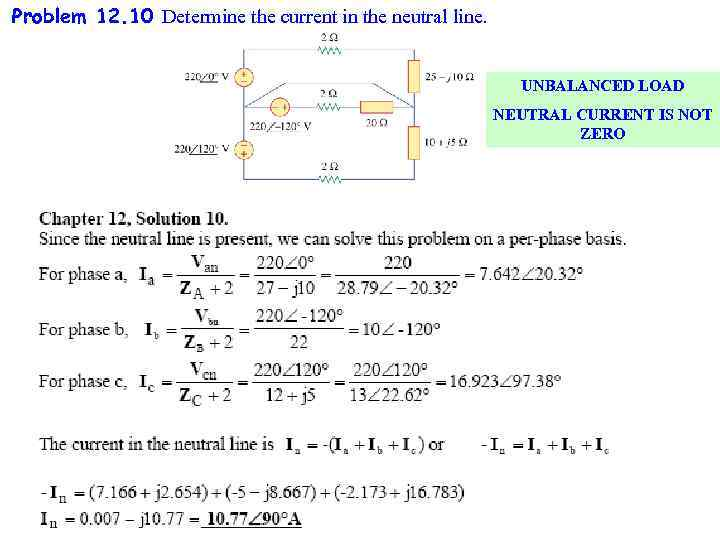Problem 12. 10 Determine the current in the neutral line. UNBALANCED LOAD NEUTRAL CURRENT IS NOT ZERO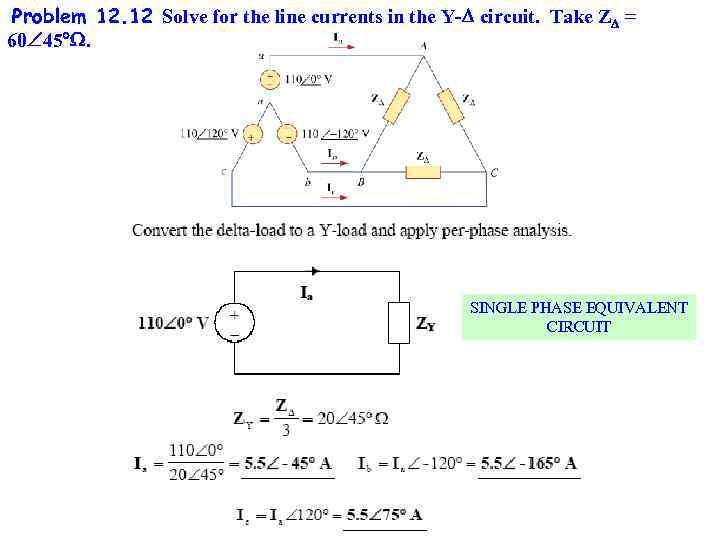Problem 12. 12 Solve for the line currents in the Y- circuit. Take Z = 60 45 . SINGLE PHASE EQUIVALENT CIRCUIT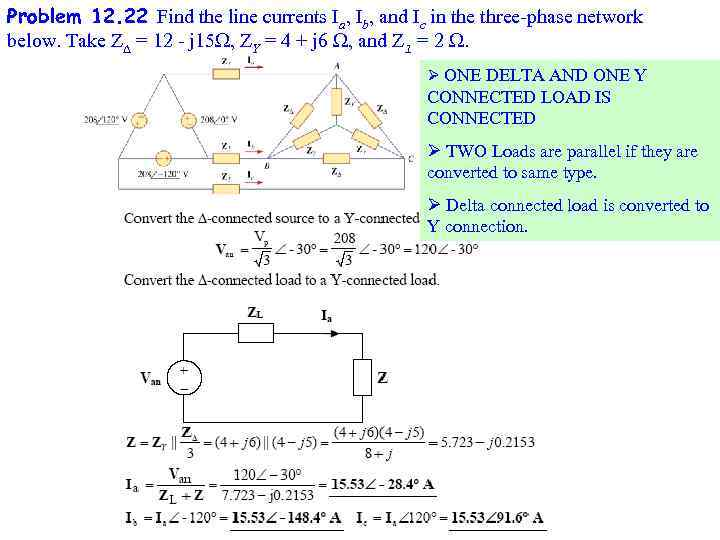Problem 12. 22 Find the line currents Ia, Ib, and Ic in the three-phase network below. Take Z = 12 - j 15 , ZY = 4 + j 6 , and Zl = 2 . Ø ONE DELTA AND ONE Y CONNECTED LOAD IS CONNECTED Ø TWO Loads are parallel if they are converted to same type. Ø Delta connected load is converted to Y connection.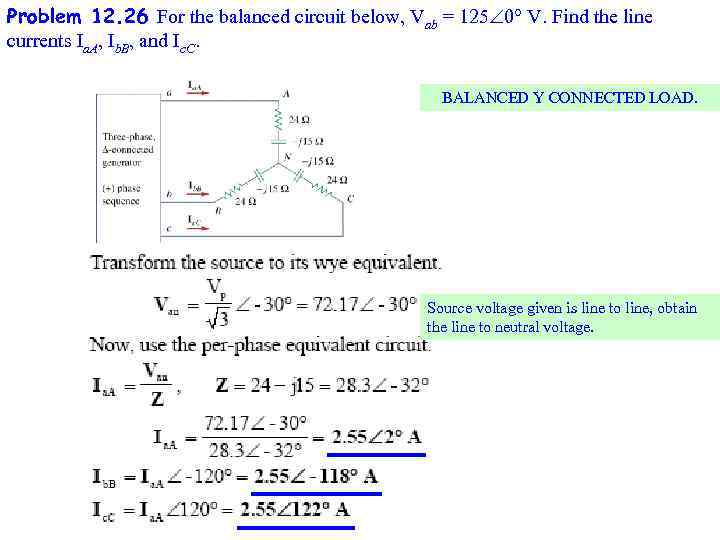Problem 12. 26 For the balanced circuit below, Vab = 125 0 V. Find the line currents Ia. A, Ib. B, and Ic. C. BALANCED Y CONNECTED LOAD. Source voltage given is line to line, obtain the line to neutral voltage.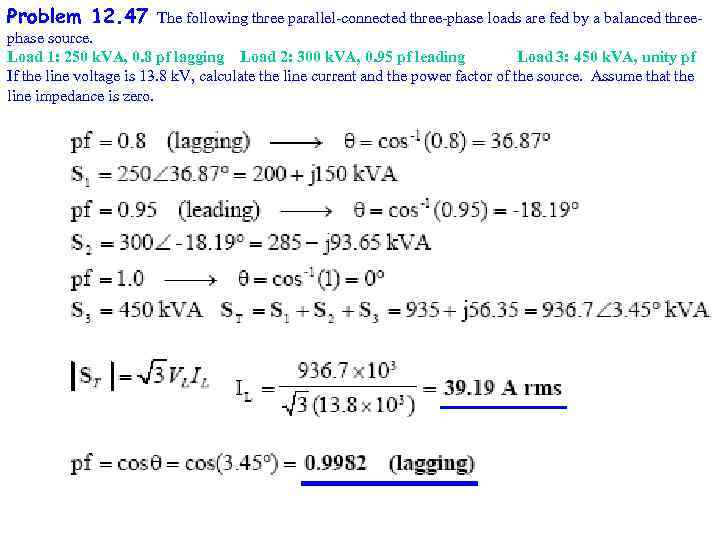Problem 12. 47 The following three parallel-connected three-phase loads are fed by a balanced three- phase source. Load 1: 250 k. VA, 0. 8 pf lagging Load 2: 300 k. VA, 0. 95 pf leading Load 3: 450 k. VA, unity pf If the line voltage is 13. 8 k. V, calculate the line current and the power factor of the source. Assume that the line impedance is zero.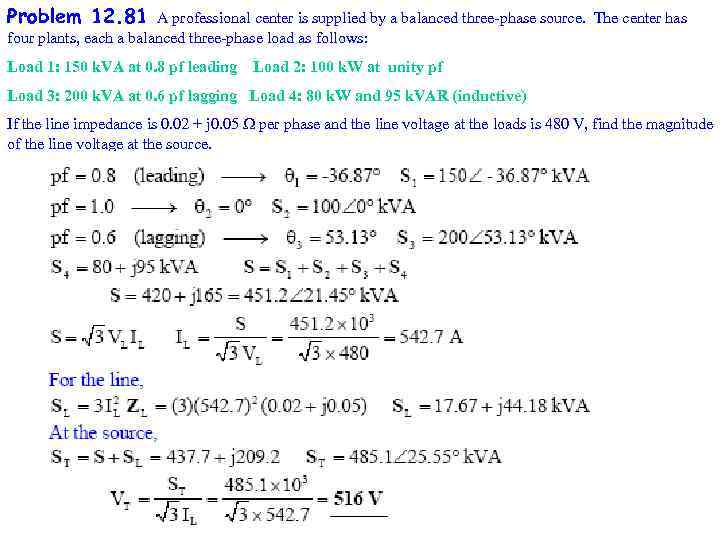Problem 12. 81 A professional center is supplied by a balanced three-phase source. The center has four plants, each a balanced three-phase load as follows: Load 1: 150 k. VA at 0. 8 pf leading Load 2: 100 k. W at unity pf Load 3: 200 k. VA at 0. 6 pf lagging Load 4: 80 k. W and 95 k. VAR (inductive) If the line impedance is 0. 02 + j 0. 05 per phase and the line voltage at the loads is 480 V, find the magnitude of the line voltage at the source.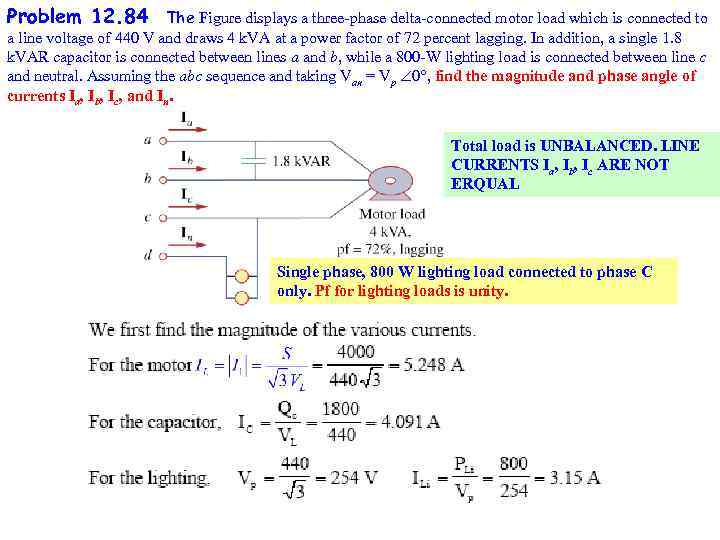Problem 12. 84 The Figure displays a three-phase delta-connected motor load which is connected to a line voltage of 440 V and draws 4 k. VA at a power factor of 72 percent lagging. In addition, a single 1. 8 k. VAR capacitor is connected between lines a and b, while a 800 -W lighting load is connected between line c and neutral. Assuming the abc sequence and taking Van = Vp 0 , find the magnitude and phase angle of currents Ia, Ib, Ic, and In. Total load is UNBALANCED. LINE CURRENTS Ia, Ib, Ic ARE NOT ERQUAL Single phase, 800 W lighting load connected to phase C only. Pf for lighting loads is unity.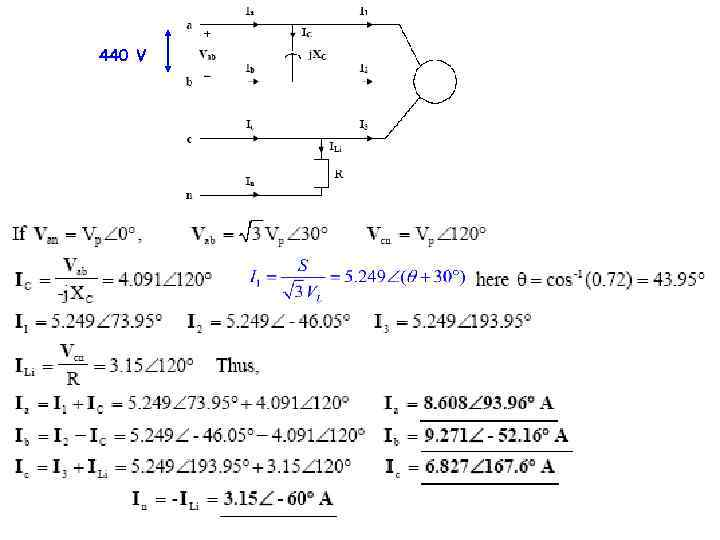440 V